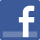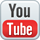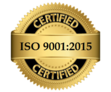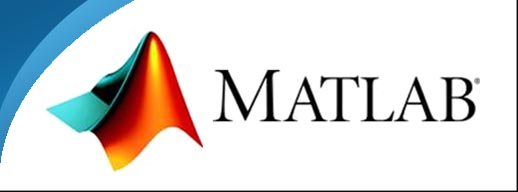﻿ Matlab computer training institue in West Delhi
• Facebook• Youtube##Professional Training & 100% Projects Based Training

Be Professionals From Professionals               Only Professionals can Make u Professional

## Overview Of MATLAB

Introduction

MATLAB or (Matrix Laboratory) is a high performance fourth generation programming language which is used for technical computing. It provides multi paradigm numerical computing environment and was developed by Math Works. It is used for integrating computation, visualization and programming so that the programming environment becomes easy to use.

MATLAB is a high-level language and interactive environment for numerical computation, visualization, and programming. Using MATLAB, you can analyze data, develop algorithms, and create models and applications. The language, tools, and built-in math functions enable you to explore multiple approaches and reach a solution faster than with spreadsheets or traditional programming languages, such as C/C++ or Java

Important Purposes

• It’s used for symbolic computation.
• Used for developing algorithm.
• Provides application of scientific and Digital-arts-courses.php graphics.
• Analysis of data, visualization, and exploration is conducted.
• Can be used for designing Graphical User Interface.
• Technical computation.

## MODULE 1

Basic elements of MATLAB

• What is MATLAB?
• Starting and stopping
• Commands in MATLAB
• Help facilities
• Arrays
• Graphs
• Script m-Files
• User defined functions

## MODULE 2

The numerical toolbox

• Introduction
• Graph of a function
• Zeroes
• Extrema
• Numerical integration
• Polynomials

## MODULE 3

The symbolic toolbox

• Introduction
• Expressions with variables
• Substitutions
• Differentiation and integration
• Numerical values
• Manipulation of expressions
• Symbols, strings and numbers

## MODULE 4

Linear algebra

• Introduction
• Matrices
• Matrix operations in MATLAB
• Solving sets of linear equations
• Numerical aspects of the use of MATLAB

## MODULE 5

Differential equations

• Differential equations
• Solving differential equations
• Solving differential equations symbolically

## MODULE 6

Programming in MATLAB

• Programming
• Writing programs
• Programming language constructs
• Creating programs
• Debugging

## MODULE 7

• Introduction
• Creating a block diagram